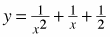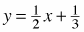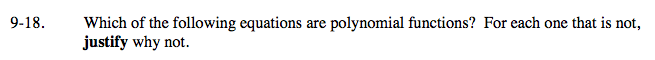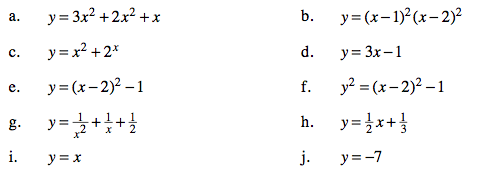### Home > A2C > Chapter 9 > Lesson 9.1.1 > Problem9-18

9-18.
1. Which of the following equations are polynomial functions? For each one that is not, justify why not. Homework Help ✎

1. y = 3x2 + 2x2 + x

2. y = (x − 1)2(x − 2)2

3. y = x2 + 2 x

4. y = 3x − 1

5. y = (x − 2)2 − 1

6. y2 = (x − 2)2 − 1

7.8.9. y = x

10. y = −7The equations in (c), (f), and (g) are not polynomials. Refer to the Math Notes box for this lesson if you are confused.